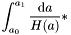# driftfac.c File Reference

compute loop-up tables for prefactors in cosmological integration More...

#include <stdio.h>
#include <stdlib.h>
#include <string.h>
#include <math.h>
#include <mpi.h>
#include <gsl/gsl_math.h>
#include <gsl/gsl_integration.h>
#include "allvars.h"
#include "proto.h"

Go to the source code of this file.

## Defines

#define WORKSIZE   100000

## Functions

void init_drift_table (void)
double get_drift_factor (int time0, int time1)
double get_gravkick_factor (int time0, int time1)
double get_hydrokick_factor (int time0, int time1)
double drift_integ (double a, void *param)
double gravkick_integ (double a, void *param)
double hydrokick_integ (double a, void *param)
double growthfactor_integ (double a, void *param)

## Detailed Description

compute loop-up tables for prefactors in cosmological integration

Definition in file driftfac.c.

## Define Documentation

 #define WORKSIZE   100000
 Referenced by init_drift_table().

## Function Documentation

 double drift_integ ( double a, void * param )
 Integration kernel for drift factor computation. Definition at line 179 of file driftfac.c.

 double get_drift_factor ( int time0, int time1 )
 This function integrates the cosmological prefactor for a drift step between time0 and time1. The value returned is *< length of the lookup table used to hold the drift and kick factors < length of the lookup table used to hold the drift and kick factors < length of the lookup table used to hold the drift and kick factors < length of the lookup table used to hold the drift and kick factors < length of the lookup table used to hold the drift and kick factors < length of the lookup table used to hold the drift and kick factors Definition at line 67 of file driftfac.c. Referenced by move_particles().

 double get_gravkick_factor ( int time0, int time1 )
 This function integrates the cosmological prefactor for a kick step of the gravitational force. < length of the lookup table used to hold the drift and kick factors < length of the lookup table used to hold the drift and kick factors < length of the lookup table used to hold the drift and kick factors < length of the lookup table used to hold the drift and kick factors < length of the lookup table used to hold the drift and kick factors < length of the lookup table used to hold the drift and kick factors Definition at line 105 of file driftfac.c.

 double get_hydrokick_factor ( int time0, int time1 )
 This function integrates the cosmological prefactor for a kick step of the hydrodynamical force. < length of the lookup table used to hold the drift and kick factors < length of the lookup table used to hold the drift and kick factors < length of the lookup table used to hold the drift and kick factors < length of the lookup table used to hold the drift and kick factors < length of the lookup table used to hold the drift and kick factors < length of the lookup table used to hold the drift and kick factors Definition at line 142 of file driftfac.c.

 double gravkick_integ ( double a, void * param )
 Integration kernel for gravitational kick factor computation. Definition at line 191 of file driftfac.c.

 double growthfactor_integ ( double a, void * param )
 Definition at line 214 of file driftfac.c.

 double hydrokick_integ ( double a, void * param )
 Integration kernel for hydrodynamical kick factor computation. < adiabatic index of simulated gas Definition at line 204 of file driftfac.c.

 void init_drift_table ( void )
 This function computes look-up tables for factors needed in cosmological integrations. The (simple) integrations are carried out with the GSL library. Separate factors are computed for the "drift", and the gravitational and hydrodynamical "kicks". The lookup-table is used for reasons of speed. < length of the lookup table used to hold the drift and kick factors < length of the lookup table used to hold the drift and kick factors < length of the lookup table used to hold the drift and kick factors < length of the lookup table used to hold the drift and kick factors Definition at line 26 of file driftfac.c. Referenced by begrun().

Generated on Sun May 22 17:33:30 2005 for GADGET-2 by1.3.9.1